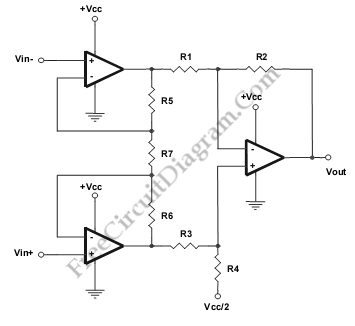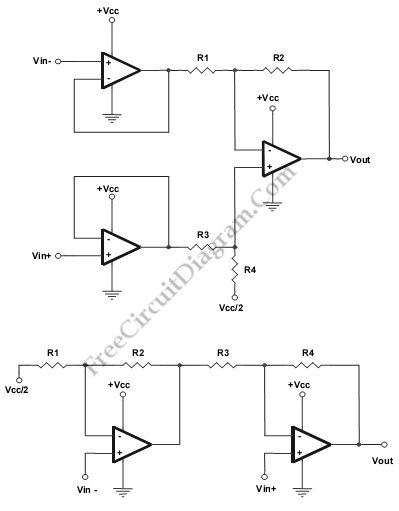# Single Supply Instrumentation AmplifierThis is a single supply instrumentation amplifier circuit. This circuit can be used to replace the function of conventional differential-amplifier topologies to load the Dc gain on a low level signal. This circuit has high input impedance of non-inverting op-amp inputs. Here is the circuit :This circuit uses single supply, so the strain gauges are operated from Vcc. There is a disadvantage of this circuit, a matched resistors are needed to build this circuit. That will suffer from poor CMRR. The circuit shown above can be modified by eliminating three resistors and by using only two op-amps. Here is the modified circuits:Two circuit above has some advantages and disadvantages. The disadvantages for first modified circuit are the two resistors must be changed instead of one and must be matched resistor. Besides that, the first stage cannnot be used for gain. However, it will easy to calculate the gain of the circuit. The second modified circuit is not recomended because it may be unstable due to the operating of first op amp at less than unity gain. Besides that the propagation delay of the signal from Vin- is more than Vin+. [Source: Texas Instruments Application Note]